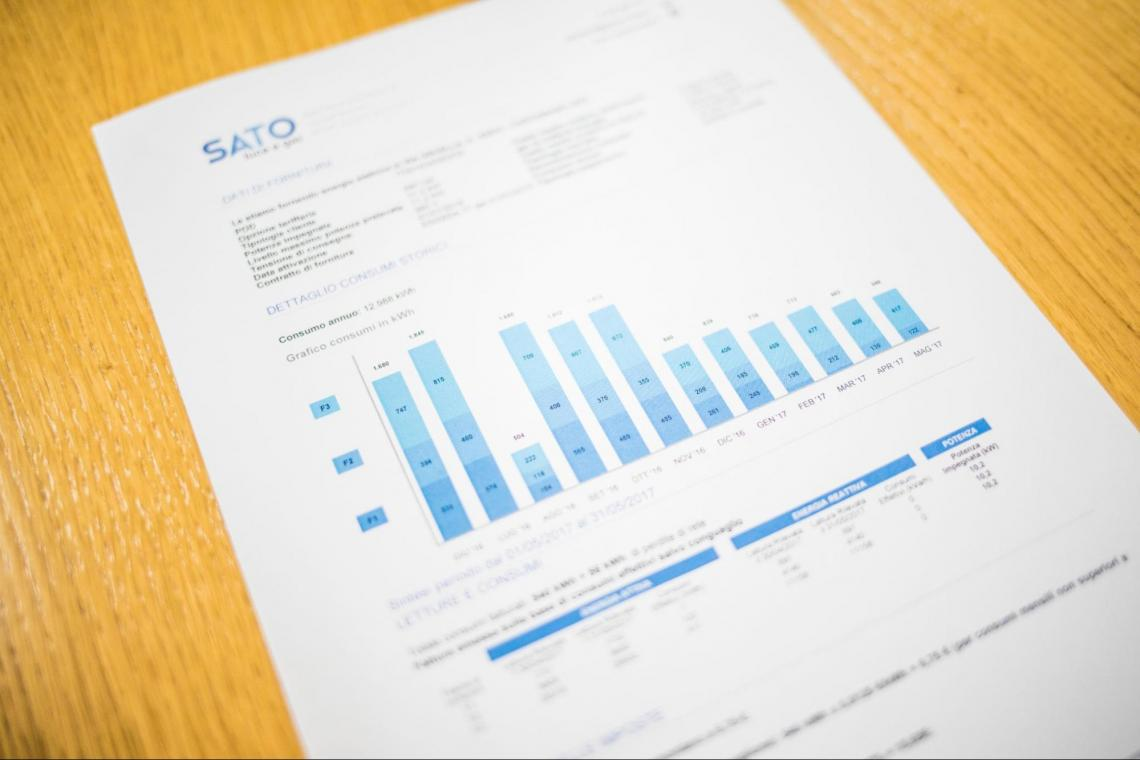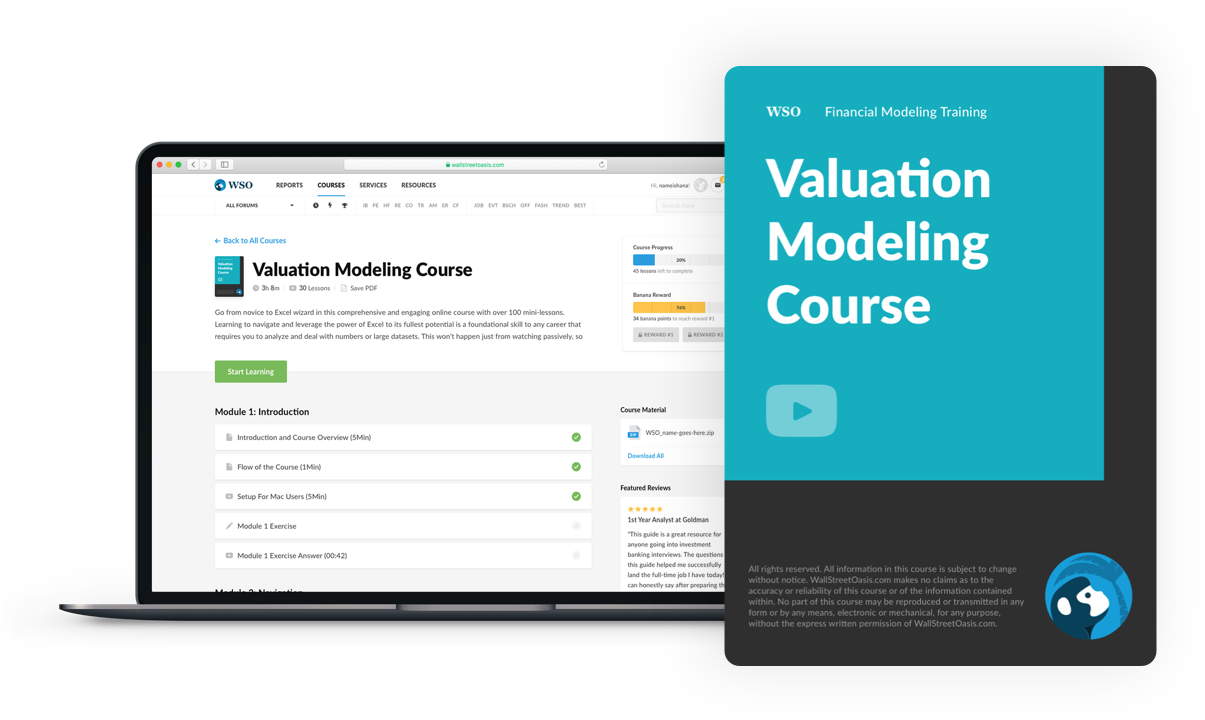# Depreciation Schedule

Charts the loss in value of an asset over its useful life.

According to your accounting method, a depreciation schedule charts the loss in value of an asset over its useful life.In the course of using up an economic asset, loss occurs. Different types of property, plants, and equipment are financial assets. As a result of usage, assets deteriorate and lose value. Foreign assets depreciate at different rates.

Utilizing a depreciation schedule simplifies the calculation.

The schedule will list the numerous asset classes, the technique each class employs, and the total depreciation that each class has accumulated throughout the schedule. This may include past and projected capital expenditures.

This article will cover all you need to know about building a plan for your business's accounting, including why a depreciation schedule is crucial, what assets you can depreciate, the various types of depreciation methods you can use, and more.

## Understanding Depreciation Schedules

Not every item a company owns is kept in a saleable state. Property, buildings, equipment, vehicles, machinery, and other fixed assets are purchased to carry out daily operating activities for a long period.Long-term assets are not fully charged when initially purchased because of their long use periods and high value. Instead, the expense is dispersed throughout their anticipated useful life. These expenses are referred to as depreciation costs in financial accounting.

The term "depreciation schedule" refers to the diagram or table used by corporations to record the costs associated with depreciating each asset over time.

You may keep track of the cost of depreciating each of your fixed assets over time by creating a table called a depreciation schedule. This schedule's main purpose is to enable you to monitor the loss you have previously identified and maintain control over your budget.

With such a schedule, businesses can monitor the long-term assets they own and predict how much they will depreciate over time.

A depreciation schedule typically contains the following details: a summary of the asset, the date of acquisition, its price, the estimated remaining life, and its value upon replacement by the company.The accelerated and straight-line methods are the two most used approaches for figuring out this cost. The straight-line method calculates an asset's value by deducting the asset's salvage value from the initial cost.

The result is divided by the projected number of useful years, and the firm deducts the same amount (the result of these calculations) each year. To reduce taxable income, an accelerated approach more quickly deducts such expenditures.

The financial statements are impacted differently depending on the methodology a company chooses. In other terms, it's crucial to consider how a corporation employs this function when examining its real bottom line.

## How to Create a Depreciation Schedule

Start by developing the following framework for the schedule. Sales revenue must be the first line item mentioned. This is because both capital expenses and such costs are frequently driven by sales revenue.Prepare a section for capital expenditures below this and include examples of prior capital expenditures. Utilizing a sound forecasting assumption, project future capital expenditures. From the following, apply your intuition to choose the appropriate forecasting assumptions:

• CapEx as a proportion of revenue or a recurrent fixed amount
• "Hard" costs that the business could reasonably anticipate incurring while it operates

1. Operation type will affect this forecast

To calculate capital expenditure as a percentage of sales when using the CapEx as a percentage of sales approach, divide CapEx by sales. Predict the percentage of sales that will be devoted to capital expenditures in the future using these percentages.

To determine a prediction for capital expenditure, multiply the result by anticipated sales.

Create a section for this expense and include any available historical depreciation expenses. You might have to infer the company's depreciation policies for such costs from previous data if you cannot ascertain them.A linear depreciation policy, such as the straight-line approach, may be used by the corporation if it appears that the expense has stayed constant. It is practical to use the expense as a proportion of net property, plant, and equipment (PP&E) in such a situation or to roll the periodic amount forward.

2. Schedule Formula

After being purchased, PP&E is a non-current (long-term) asset that continues to benefit the business for the period of its useful life. It estimates how long the asset will be of service to the business.

The value of an asset is lost over the expected years of its useful life. Depreciation is the distribution of a one-time capital expenditure cash outflow throughout a fixed asset's useful life. The goal is to reduce balance-sheet asset value while retaining the asset's ability to generate revenue.Even though depreciation is added back to the cash flow statement as a non-cash item, the fact that it is tax deductible means that the expense lowers the period's tax burden.

As a non-cash expense frequently impacted by the firm's discretionary assumptions, devaluation is a crucial factor to consider when establishing a company's underlying cash flow profile (i.e., determining the useful life).

Due to the "noise" depreciation frequently causes when analyzing net income as reported on the income statement, the cash flow statement is also required to assess a company's success.

## Accelerated Depreciation Schedule vs. Straight-Line Depreciation

The most popular methodology is known as "straight-line" depreciation. Using this strategy, the value of PP&E on the balance sheet is reduced steadily until the residual value is zero.When an asset reaches the end of its useful life, its salvage value is determined. Practically speaking, salvage value can be understood as the price at which a business can sell an outdated asset at the end of its useful life; frequently, this amounts to the value of the parts.

The vast majority of businesses make use of the salvage value assumption, according to which the asset's residual worth is reduced to zero by the time its useful life is up.

Suppose the salvage value is assumed to be zero. In that case, the result is that the depreciation expense per year will be larger, and the depreciation tax benefits will be completely realized (this is the common assumption used in straight-line depreciation).

Under straight-line depreciation, the depreciation cost for a \$100k purchase of PP&E with an estimated useful life of 5 years would be \$20k annually.

As a result, \$100k in PP&E was bought at the end of the first period (Year 0), and its value on the balance sheet will drop by \$20k per year until it hits zero at the end of its useful life (Year 5).

Other methods of calculating depreciation exist, most notably, accelerated accounting, which accelerates the asset's depreciation in its initial years.

This practice thus reduces net income earlier on than it does later. However, the majority of publicly traded corporations choose straight-line depreciation due to how much they value their net income and earnings per share (EPS) figures.

Because straight-line depreciation will result in fewer losses being recorded in the initial years of an asset's useful life than under accelerated loss, most businesses prefer it for GAAP reporting purposes.Companies often prioritize short-term financial performance. This is why the accelerated depreciation technique makes net income and EPS initially lower and only higher in the following years and is unattractive to many businesses.

The idea behind accelerated loss is that, as the item loses more value earlier in its lifecycle, greater deductions can be made. Further into the asset's useful life, the accelerated technique ultimately starts to appear on the income statement with reduced devaluation.

However, to underline, businesses still favor straight-line devaluation due to timing. Ultimately, the total devaluation amount and timing of the cash outflow are the same, but the impact on net income and EPS for reporting purposes is different.

## Summary

Publish a breakdown of the net change in PP&E at the bottom of the depreciation schedule. Starting with the PP&E balance at the beginning of the period, add capital expenditures and deduct depreciation expenses.

Also, deduct any sales or write-offs from the starting balance. The ending balance of PP&E, already net of cumulative evaluation, should be used to calculate the final amount.

It may be wise to smooth the projections at this time. For example, consider reviewing the forecasting assumptions for each item if the trend in the future appears to be erratic or if the link between future CapEx and depreciation expense diverges. This is important in the real estate sector.### Everything You Need To Master Valuation Modeling

To Help You Thrive in the Most Prestigious Jobs on Wall Street.

The devaluation plan is utilized to track the amassed depreciation and remaining value of a settled resource based on its useful life assumption.  Depreciation is a non-cash cost that decreases the book value of a company's property, plant, & equipment over its lifespan.

Under U.S. GAAP, devaluation is an accounting method used to adjust the timing of settling asset purchasing with money streams created from these long-term resources.

Create a depreciation schedule based on the company's current PP&E and total PP&E purchases for project devaluation as an alternative method.

According to this method, new CapEx must be projected using management's useful life assumptions and the average remaining useful life for existing PP&E.

Consider revisiting the forecasting assumptions for each component if future trends appear rough or if future depreciation expense is not closely correlated. Depreciation schedules are extensively used in the real estate industry due to their unique nature.### FAQs### Everything You Need To Build Your Accounting Skills

To Help You Thrive in the Most Flexible Job in the World.

Researched and authored by Drishti Kohli | LinkedIn

Reviewed and edited by James Fazeli-Sinaki | LinkedIn